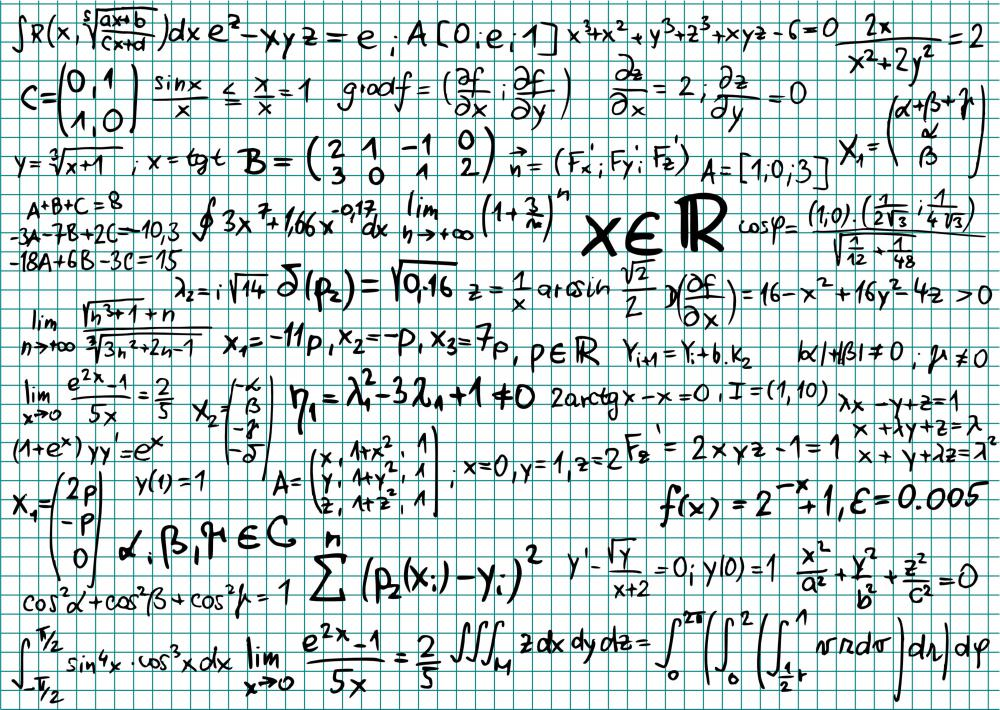# What Is the Arithmetic Mean?

Jennifer Voight

The arithmetic mean is a measure of central tendency calculated by adding the values of all numbers within a set and dividing the total by the quantity of items in the set. All numbers in the set must be positive, real numbers. The terms average and mean also refer to the arithmetic mean and are more commonly used in real-life situations.The arithmetic mean is calculated by adding the values of all numbers within a set and dividing the total by the number of items in the set.

Distinct from the values of the geometric mean and harmonic mean, the arithmetic mean is always greater than or equal to the geometric mean. The geometric mean is always greater than or equal to the harmonic mean, when only real, positive numbers are used. Together, arithmetic mean, geometric mean, and harmonic mean are referred to as the three Pythagorean means.

When the lowest number and highest number in a set are compared with the arithmetic mean of a set, the mean will always lie in between the lowest and highest numbers. The mean does not always lie in the middle of the set of numbers, however. This is because it can be greatly affected by the presence of either extreme high values or extreme lows, also called outliers. For this reason, there are are other measures of central tendency, like mean and mode, to help describe a set.

An example is a set whose values are 4, 6, 7, 10, 13, and 34. The mean equals 12.3, which is more than a person's sense of where the middle might be. Yet when one value, 34, is changed to 14 to more closely match the others, the arithmetic mean is 9. Despite its weaknesses, the arithmetic mean is commonly used in most academic fields other than statistics and mathematics, especially economics, social science, and history.

When dealing with the arithmetic mean, half of the values must be higher than the average of a set, while the other half of the values must be lower than the mean. This does not apply to the number of items in the set. The arithmetic mean acts as the fulcrum of a balance for the values.

Although the arithmetic mean is a commonly understood concept that is easy to calculate, there are situations when the geometric mean or harmonic mean provides more accurate information about a set of values. Frequently, harmonic mean has applications to engineering data, especially when determining means of rates. Geometric mean can be descriptive of economic data, proportional growth, or social science statistics.

## You might also Like